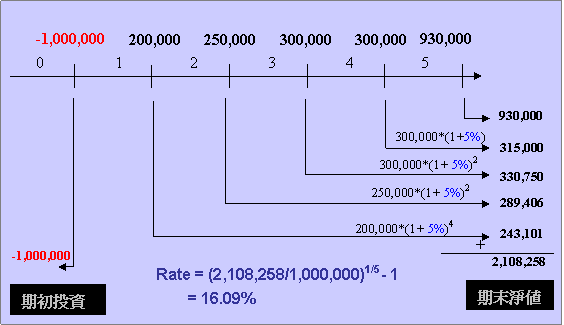# 評估投資的好工具

﻿

## 淨現值(NPV)

PV = C1 / (1+R)1 + C2 / (1+R)2 + ….. + Cn / (1+R)n ------------- (1)

NPV = PV – C0 ------------------------(2)

• 第一年報酬20萬
• 第二年報酬25萬
• 第三年報酬30萬
• 第四年報酬30萬
• 第五年報酬加頂讓共得款93萬

NPV = 200,000/(1+20%)1 + 250,000/(1+20%)2+ 300,000/(1+20%)3+ 300,000/(1+20%)4+ 930,000/(1+20%)5 – 1,000,000

= 200000/(1+20%)^1+ 250000/(1+20%)^2+ 300000/(1+20%)^3+ 300000/(1+20%)^4+ 930000/(1+20%)^5 -1000000 = 32,311NPV = 20000000/(1+10%)^1+20000000/(1+10%)^2+20000000/(1+10%)^3+20000000/(1+10%)^4+20000000/(1+10%)^5+20000000/(1+10%)^6-100000000 = -12,894,786

Excel的NPV函數不是淨現值

Excel有提供一個NPV函數，但是意義卻不是真正淨現值，這函數是計算所有現金流量現值的加總。例一的淨現值如果直接用Excel 的NPV函數代入，是會得到錯誤的結果。

=NPV(20%,-1000000,200000,250000,300000,300000,930000) = 26,925

=-1000000 + NPV(20%,200000,250000,300000,300000,930000) = 32,311

## 內部報酬率(IRR)

NPV = 200,000/(1+R)1 + 250,000/(1+ R)2+ 300,000/(1+ R)3+ 300,000/(1+ R)4+ 930,000/(1+ R)5 – 1,000,000

0 = 200,000/(1+R)1 + 250,000/(1+ R)2+ 300,000/(1+ R)3+ 300,000/(1+ R)4+ 930,000/(1+ R)5– 1,000,000

=IRR({-1000000,200000,250000,300000,300000,930000}) = 21.14%R = (FV/PV)1/n -1 -----------(3)

FV =200000*(1+21.14027%)^4+ 250000*(1+21.14027%)^3 + 300000*(1+21.14027%)^2 + 300000*(1+21.14027%)^1+ 930000*(1+21.14027%)^0 = 2,608,811

## 修正投資報酬率(MIRR)

=200000*(1+5%)^4+ 250000*(1+5%)^3 + 300000*(1+5%)^2 + 300000*(1+5%)^1+ 930000*(1+5%)^0

= 2,108,257.5=MIRR({-1000000,200000,250000,300000,300000,930000}, 8%, 5%)
= 16.09%

### 資金利率(Finance Interest Rate)

{-1000000, 200000, -250000, 300000, 300000, 930000}

=250000/(1+8%)^2 = 214,334.7

MIRR計算原則如下：將所有的現金流入(正值)以在投資利率rrate求取未來值加總就是期末淨值FV；將所有的現金流出(負值)以資金利率frate求取現值加總就是期初總投入PV，再利用式子(3)求R值。

=1000000+250000/(1+8%)^2 = 1,214,334.7

=200000*(1+5%)^4+ 300000*(1+5%)^2 + 300000*(1+5%)^1+ 930000*(1+5%)^0 = 1,818,851.2

=(1818851.2/1214334.7)^(1/5)-1= 8.416%=MIRR({-1000000,200000,-250000,300000,300000,930000}, 8%, 5%) = 8.416%

# 其他網站之參考資料

﻿

■ 本網站內容儘可能精確完整，但不保證無誤。若做為投資依據，風險請自行斟酌 ，本網站不負賠償之責任。
■ 網站所有資料均為版權所有，非經書面允許請勿轉載或使用。